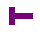# Self printing signature programs

Signature programs are short programs that people have been using as part of their signature. They are small (2 to 4 lines) programs, that are often rather cryptic because they have to save space as much as possible. On this page some self printing signature programs are presented. Self printing programs are also called Quines.

## Dario Dariol

Below are three self printing programs in C written by Dario Dariol, which are described in the standard GNU distribution since 1990:
```main(a,b){a="main(a,b){a=%c%s%c;b='%c';printf(a,b,a,b,b);}";b='"';printf(a,b,a,b,b);}
main(a){a="main(a){a=%c%s%c;printf(a,34,a,34);}";printf(a,34,a,34);}
main(a){printf(a="main(a){printf(a=%c%s%c,34,a,34);}",34,a,34);}
```
Only the first version is ASCII independent. The first two are compiler independent. Dario also wrote a hiding siganture programs and a self-printing one in Java.

## Mike Davis and Paul Makepeace

Another self printing program in C is:

```char *p="char *p=%c%s%c;main(){printf(p,34,p,34);}";main(){printf(p,34,p,34);}
```
This has been used by Mike Davis ..!att!ihlpm!jmdavis, and Paul Makepeace.

## By Maarten Fokkinga using Miranda

A shorter one, in Miranda by Maarten Fokkinga:
```t c=c++show c;self=s"t c=c++show c;self=s"
```

## Tom Phoenix using Korne shell

An this one, which is even shorter, works for Tom Phoenix on his Unix system:
```% /bin/ksh: /bin/ksh::  not found
```

## Teemu Suikki

```main() {char *p,a;printf(p="main() {char *p,a;printf(p=%c%s%c,a='",a='"',p,
a);printf(p="%c',p,%ca);printf(p=%c%s%c%c,a,10,a,p,a,10,a,%c%s%c,a,10);}%c"
,a,10,a,p,a,10,a," *** Email: xxxxxxx@xxx.xx *** IRC: Zuikkis *** ",a,10);}
```

## A self printing Perl signature program by Dimator Shahbaz

```\$_=q{\$_=q{Q};s/Q/\$_/;print};s/Q/\$_/;print
```

## Self printing Java signature program by Dario Dariol

A little long (444 characters) for a signature, but as the first Java attempt, worth mentioning:
```import java.text.*;class a{public static void main(String x[]){char b[]={34};char c[]={123};String s[]=new String;s="import java.text.*;class a{2}public static void main(String x[]){2}char b[]={2}34};char c[]={2}123};String s[]=new String;s={1}{0}{1};s=new String(b);s=new String(c);System.out.println(MessageFormat.format(s,s));}}";s=new String(b);s=new String(c);System.out.println(MessageFormat.format(s,s));}}
```
He also wrote some in C and a hiding program signature program.

## Self printing Python signature program by BReflection

```python -c "x='python -c %sx=%s; print x%%(chr(34),repr(x),chr(34))%s'; print x%(chr(34),repr(x),chr(34))"
```

Signature programs | home and email
```

```